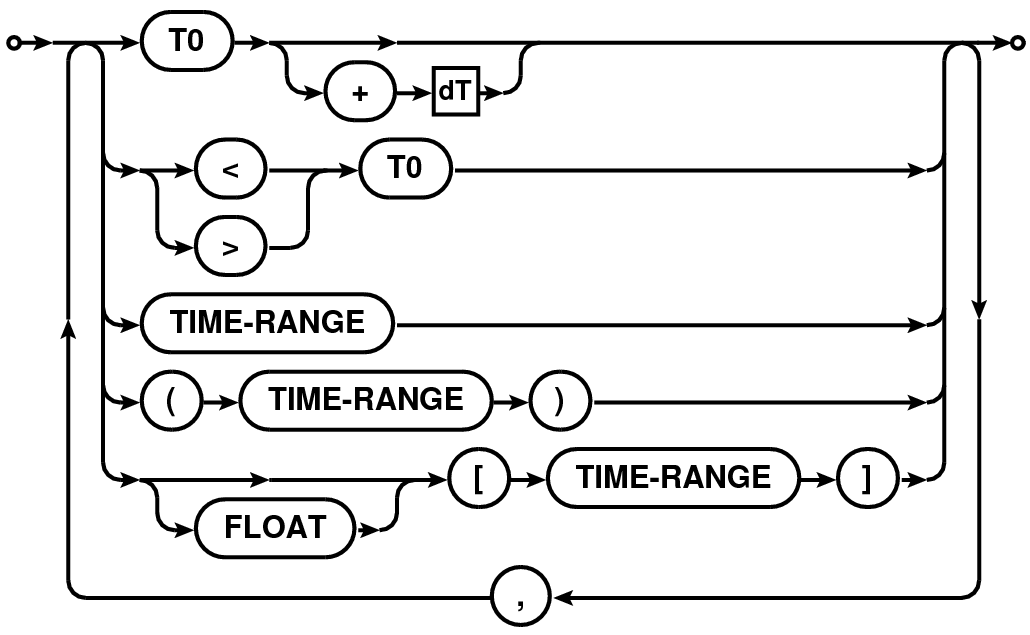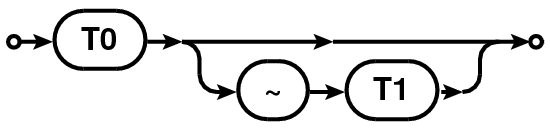2 Time Selection

Time selection expression is a comma separated list of time specifications. Time can be specified in the format YYYY/MM/DD/HH:MM:SS.FF. Fields (i.e., YYYY, MM, DD, HH, MM, SS and FF), starting from left to right, can be omitted. Omitted fields will be replaced by context sensitive defaults as explained below.

Table 1: Railroad diagram for the syntax of time/time-range selection expression.

 Syntax of Railroad Diagram TIME ExpressionTIME-RANGE2.1 Syntax

Various forms of possible time-selection expression are listed below. T0, T1 and dT in the following description are time specifications.

• time =  T0~T1

Selects all time stamps which satisfy the following condition:

(TimeStamp >= T0) and (TimeStamp <= T1)

Fields missing in T0 are replaced by the fields in the time stamp of the first valid selected row in the MS. Fields missing in T1 are replaced by the corresponding fields of T0 (after its defaults are set).

• time =  [T0~T1]

The edges of the range is computed using the Exposure Time. All rows with a time stamp which satisfy the following condition are selected:

((TimeStamp > T0) or (abs(TimeStamp - T0) < ExposureTime/2)) and
((TimeStamp < T1) or (abs(TimeStamp - T1) < ExposureTime/2))

Fields missing in T0 are replaced by the fields in the time stamp of the first valid selected row in the MS. Fields missing in T1 are replaced by the corresponding fields of T0 (after its defaults are set).

• time =  FLOATNUMBER [T0~T1]

Same as the previous expression with the edges of the range determined using the FLOATNUMBER (in seconds of time) instead of Exposure Time. All rows with a time stamp which satisfy the following condition are selected:

((TimeStamp > T0) or (abs(TimeStamp - T0) < FLOATNUMBER)) and
((TimeStamp < T1) or (abs(TimeStamp - T1) < FLOATNUMBER))

Fields missing in T0 are replaced by the fields in the time stamp of the first valid selected row in the MS. Fields missing in T1 are replaced by the corresponding fields of T0 (after its defaults are set).

• time =  T0

All rows with time stamp that is within an integration time of T0 are selected. Integration time is determined from the first valid selected row (more rigorously, an average integration time should be computed). Default settings for the missing fields of T0 are as described in bullet (1) above.

• time =  T0+dT

All rows with time stamp starting from T0 and ending with time value given by T0+dT are selected. Defaults of T0 are set as usual described in bullet (1) above. Defaults for dT are set from the time corresponding to MJD=0. I.e. dT is an specification of length of time from nominal ”start of time” (the time stamp of the first valid selected row of the MS).

• time =  >T0

Selects all times greater than T0.

• time =<T0

All rows with time stamp less than T0 are selected. Default settings for T0 are as above.